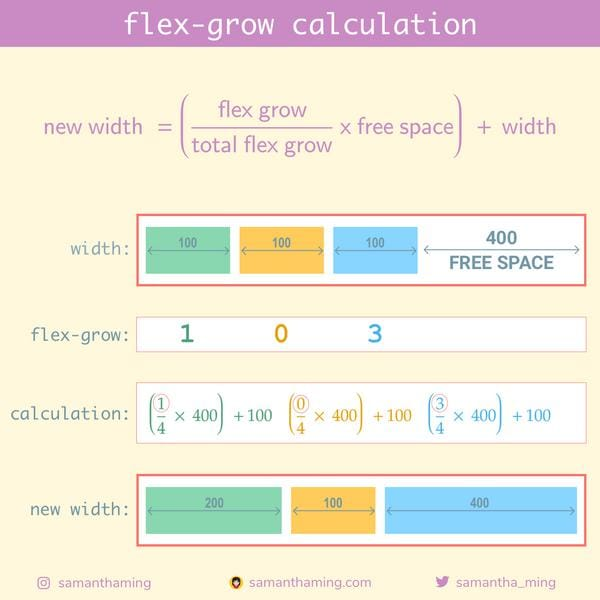# # Day 22: flex-grow calculation

Being able to grow and fill the free space is pretty cool. Because we don't set the final width of our flex item, the size it grows to always seem so random to me. So let's look at the math. Honestly you don't need to know this to understand Flexbox. The browser takes care of this automatically for you. But knowing what's behind this sorcery might demystify this process and help you understand it better. It's like once you know the trick to the magic, you're no longer tricked by the magic 😉## # Calculation

I know it can be quite overwhelming to see all numbers crammed into a tidbit. So let's walk through the calculation 👍

Here's the `HTML` and `CSS` we're working with:

HTML

``````<div class="parent">
<div class="child green"></div>
<div class="child yellow"></div>
<div class="child blue"></div>
</div>
``````

CSS

``````.parent {
width: 700px;
}
.child {
width: 100px;
}
.green {
flex-grow: 1;
}
.yellow {
flex-grow: 0;
}
.blue {
flex-grow: 3;
}
``````

### # Step 1: Breaking down the variables

Here's the formula:

``````new width = ( (flex grow / total flex grow) x free space) + width
``````

Let's extract the variables required in the formula to this handy table we can fill in as we go:

Variables
flex grow provided from css
total flex need to calculate
free space need to calculate
width provided from css

### # Step 2: Fill in what we know

From the `CSS` value, we can conclude the following:

• Each child element has a width `100`
• The parent element (container) has a width of `700`
• The child has a `flex-grow` of `1`, `0`, `3`

Let's update our chart with this information:

Green Yellow Blue
flex grow 1 0 3
total flex
free space
width 100 100 100

### # Step 3: Calculate "free space"

This is the formula:

``````free space = parent width - total children widths
``````

Remember what we know:

• Each child element has a width `100`
• The parent element (container) has a width of `700`

Great, we can use that information to calculate "total children widths":

``````total children widths = green + yellow + blue
= 100   + 100    + 100

=> 300
``````

Now we can calculate our "free space":

``````free space = parent width - total children widths
= 700          -  300

=> 400
``````

Green Yellow Blue Total
flex grow 1 0 3
total flex
free space - - - 400
width 100 100 100

### # Step 4: Calculate "total flex grow"

This is an easy one, we simply add up our total `flex-grow`:

``````total flex grow = green + yellow + blue
= 1     + 0      + 3

=> 4
``````

Fill in our chart and Voilà! We have all the information we need for the final calculation 👍

Green Yellow Blue Total
flex grow 1 0 3 4
free space - - - 400
width 100 100 100

### # Final step: Calculate "new width"

Remember the formula:

``````new width = ( (flex grow / total flex grow) x free space) + width
``````

a. Green

``````new width = ( (1/4 * 400) ) + 100

=> 200
``````

b. Yellow

``````new width = ( (0/4 * 400) ) + 100

=> 100
``````

c. Blue

``````new width = ( (3/4 * 400) ) + 100

=> 400
``````

Done! We have successfully calculated the new width 🥳

Green Yellow Blue Total
width 100 100 100
flex grow 1 0 3 4
free space 400
new width 200 100 400